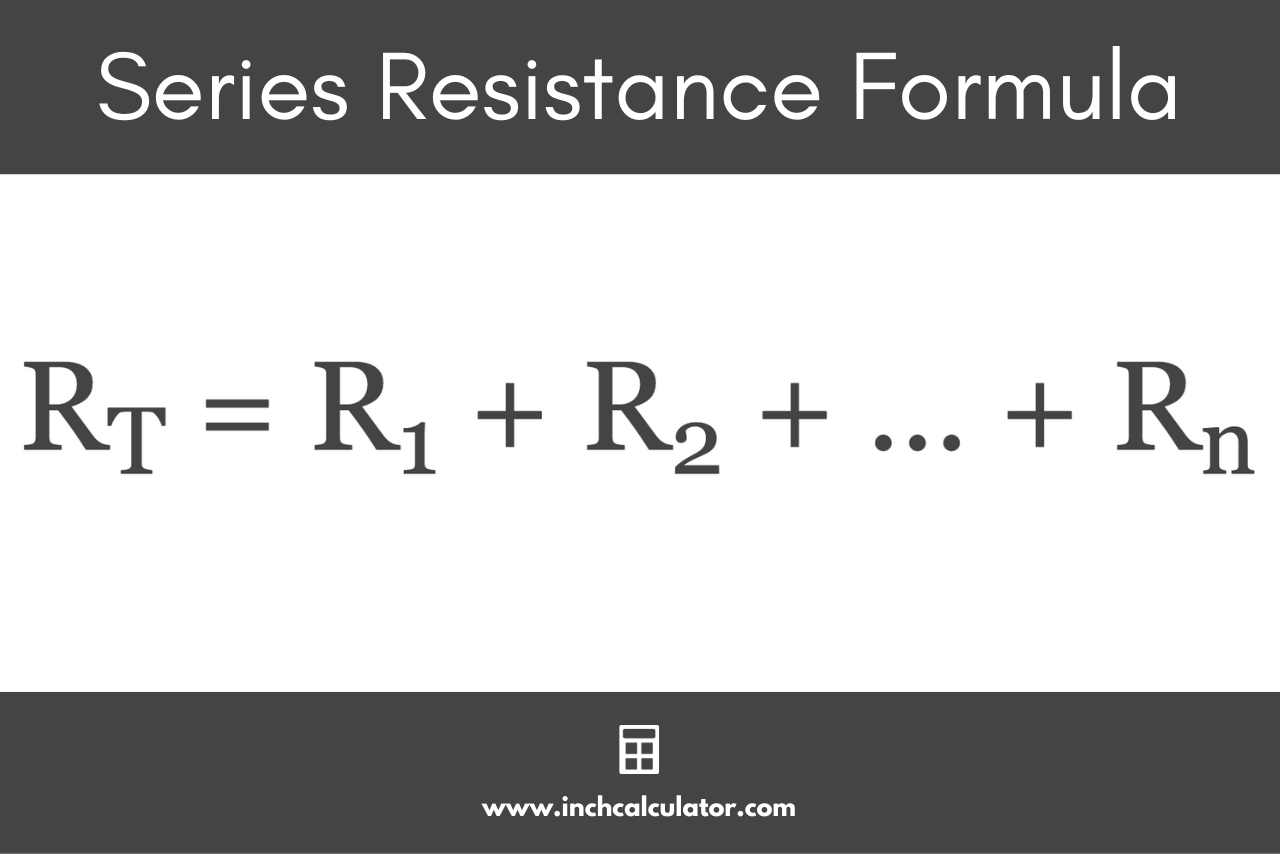# Series Resistor Calculator

Calculate the resistance in ohms of resistors connected in series by entering the value of each.

## Series Resistance:

Learn how we calculated this below

Do you want to calculate resistors in parallel?

## How to Calculate Resistors in Series

Resistors are electrical components that add resistance to a circuit. This calculator finds the total resistance measured in ohms (Ω).

Resistors connected in series are connected from one to the next, and current flows through each of them in the line.

There is a single path for current to flow, and because of this, finding the total resistance of resistors connected in series can be done by summing the values.

### Series Resistor Formula

When multiple resistors are connected in series the total resistance can be found using this formula.

RT = R1 + R2 + … + Rn

So, the total resistance of resistors connected in series is equal to the value of each added together.For example, let’s find the total resistance of a circuit with 200 ohm, 300 ohm, and 400 ohm resistors connected in series.

RT = 200 Ω + 300 Ω + 400 Ω
RT = 900 Ω